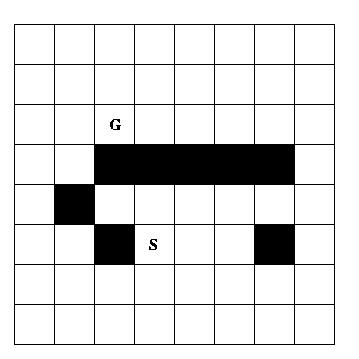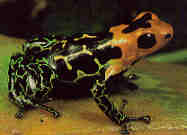## Assignment1 COSC 6368: Heuristic Search

Assigned: 8/28/02
Due: 10/1/02 (Problems 1-4)
Due: 10/8/02 (Problem 5)
Last Updated: 9/16/02
Value: 120 (1: 12, 2:12, 3:12, 4:12, 5: 72)

Remark: Problems 1-4 are paper and pencil problems, whereas problem 5 involves some programming and experimentation.

### Problem 1 --- State-Space Search Representation

The "3 Missionaries and 3 Cannibals Problem" is defined as follows. Three missionaries and three cannibals are standing on the left bank of a river. There is a small boat to ferry them across with enough room for only one or two persons. Exactly one or two persons must be in the boat each time it crosses the river in either direction. An indivisible action corresponds to one or two people getting into the boat, moving across the river, and then all passengers disembarking the boat. The goal is to get all 6 people across the river. If ever there are more missionaries than cannibals on either side of the river, the missionaries will convert the cannibals. Find a sequence of crossings to transport safely all the missionaries and cannibals across the river without exposing any of the cannibals to conversion.

(a) Formulate this problem as a state-space search problem Give a precise definition of a state, the start state, the goal state or goal condition, and the operators. Operators should be specified as "schemas" that indicate for a general state, when the operator is legal (i.e., a set of constraints on when the operator can be applied to an arbitrary state, which is given as an n-tuple) and the description of the successor state (given as an n-tuple that is completely specified from the previous state's n-tuple and the parameters associated with the given operator) after the operator is applied. In other words, each operator should be specified in a way that it would easily implemented in a program to solve this problem.

(b) Show the State Space Draw the complete state-space graph that includes all nodes (and legal directed arcs connecting these nodes) for this problem. Inside each node show the state description, and label each arc with its associated operator. Highlight a path that gives a solution to the problem.

### Problem 2 --- Comparing Multiple Search Methods

Consider the problem of finding a path in the grid shown below from starting square S to goal square G. Possible moves from a square are to move up, left, right, and down exactly one square. No move may be made onto a dark square (i.e., obstacles) or off the edge of the grid.

(a) Depth-First Search
Copy the grid and then mark the grid squares with the number(s) indicating when that square is expanded during a Depth-First search from S to G. Assume children nodes are visited in the order up, left, right, down. Assume cycle checking is done so that a node is not generated in the search tree if the grid square position associated with the node occurs somewhere on the path from this node back to the root node. Highlight the solution path found, if any, or explain why none is found.

(b) Greedy Best-First Search
Copy the grid again and then mark the grid squares with the number(s) indicating when that square is expanded during a Greedy Best-First search from S to G. Use as the heuristic function h(n) = |xn - xg| + |yn - yg| where the grid square associated with node n is at coordinates (xn, yn) in the grid and the goal node G is at coordinates (xg, yg). Assume you do not generate a node if that node's associated grid position has previously been generated. (That is, the third way to deal with repeated states as described on page 82.) In the case of ties in evaluation function values, for siblings expand them in the precedence order up, left, right, down. In the case of ties between non-siblings, use FIFO order to expand first the node that has been in the NODES list the longest. Highlight the solution path found, if any, or explain why none is found.(c) A* Search
Copy the grid again and do the same as in (b) except using A* search with the same heuristic function as in (b) and the cost of each move equal to 1. Use the same tie-breaking rules as defined in (b). Highlight the solution path found, if any, or explain why none is found.

(d) Hill-Climbing Search
Copy the grid again and use Hill-Climbing Search. As above, number the squares when a node is expanded. Use the heuristic function defined in (b). Use the same tie-breaking rules as defined in (b). Highlight the solution path found, if any, or explain why none is found.

(e) An Infinite Grid
Suppose the grid is extended infinitely in all directions but S, G and the dark squares remain as before. Which of the following methods will not be able to find a solution to this problem? Breadth-First, Depth-First, Depth-First Iterative Deepening, Greedy Best-First, and A*. Which of these would be the best method, and why? Again, in the case of ties in ordering nodes on the NODES list, use the precedence defined in (b).

### Problem 3 --- Admissible Heuristics

If h1(s) and h2(s) are both admissible heuristic functions, which of the following are also admissible:

1. h3(s) = h1(s) + h2(s)
2. h3(s) = | h1(s) - h2(s) | (The ||'s indicate ABSOLUTE VALUE.)
3. h3(s) = max(h1(s), h2(s))
4. h3(s) = min(h1(s), h2(s))

Be sure to explain your answers. For those cases where h3(s) is not admissible, show a counter example.

Which of the above four combinations do you feel is the best one? Why?

### Problem 4 --- More Heuristic Search

(a) Assume a non-finite maze is given in which a robot has the task to move from an initial position (0,0) to a goal position. The available operators are north, south, east, and west that move the robot one field in the indicated direction. However, sometimes the robot cannot move in a particular direction e.g. if the robot faces a wall in the north it cannot move north. In the particular problem we try to reach one of 2 goal positions (100,300) and (300,100); unfortunately, usually, one of the two goal positions is unreachable.

```(0,0) (0,1) (0,2) (0,3) (0,4)…
(1,0) (1,1) (1,2) (1,3) (1,4)…
(2,0) (2,1) (2,2) (2,3) (2,4)…
(3,0) (3,1) (3,2) (3,3) (3,4)…
(4,0) (4,1) (4,2) (4,3) (4,4)…
```

Assume best-first search is used for this particular problem, and the objective is to find a path to the reachable goal position. Three evaluation functions, listed on the next page, are used to solve this problem (assume that states with the lowest values will be expanded first). For each of the three evaluation functions answer the following two questions: If the evaluation function is used will best-first always find a solution? If your answer to the first question is yes, also evaluate the efficiency of the search! Give reasons for your answers!

1) h1(x,y):= |x|+|y|

2) h2(x,y):= if x< 200 then |x-100| + |y-300|
else if x<400 then |x-300| + |y-100|
else 10000

3) h3(x,y):= min(|x-100| + |y-300|, |x-300| + |y-100|)

(b) Now assume you have to use backtracking for the above problem. Is backtracking a suitable search strategy for the problem? Give reasons for your answer!

### Problem 5 --- Hill Climbing and Simulated Annealing (Programming Problem)

Write a program that is capable of solving optimization (minimization) problems involving functions functions of arity 3 f(a,b,c) with a,b,c e[0,1] using

(1) randomized hill climbing

(2) simulated annealingDesign your program in such a way that parameters for each techniques (size of a neighborhood, number of samples in a neighborhood for hill-climbing; size of neighborhood, cooling schedule,... in the case of simulated annealling) can be selected (or propose a technique that automatically selects/finds the "best parameter" setting during the run). Run your program for the 3 functions of a benchmark that is provided to you in the third week of September also trying to find the best parameter settings of each technique for each function. Write a short (2-4 single space pages) report that explains the capabilities and interfaces of a program and techniques that your program uses. Moreover, your report should also compare the two techniques for the 3 optimization problems in the benchmark. Be also prepared to demo your program. Moreover, add a simple visualization component to your program that visualizes the search your program conducts for a particular optimization problem.